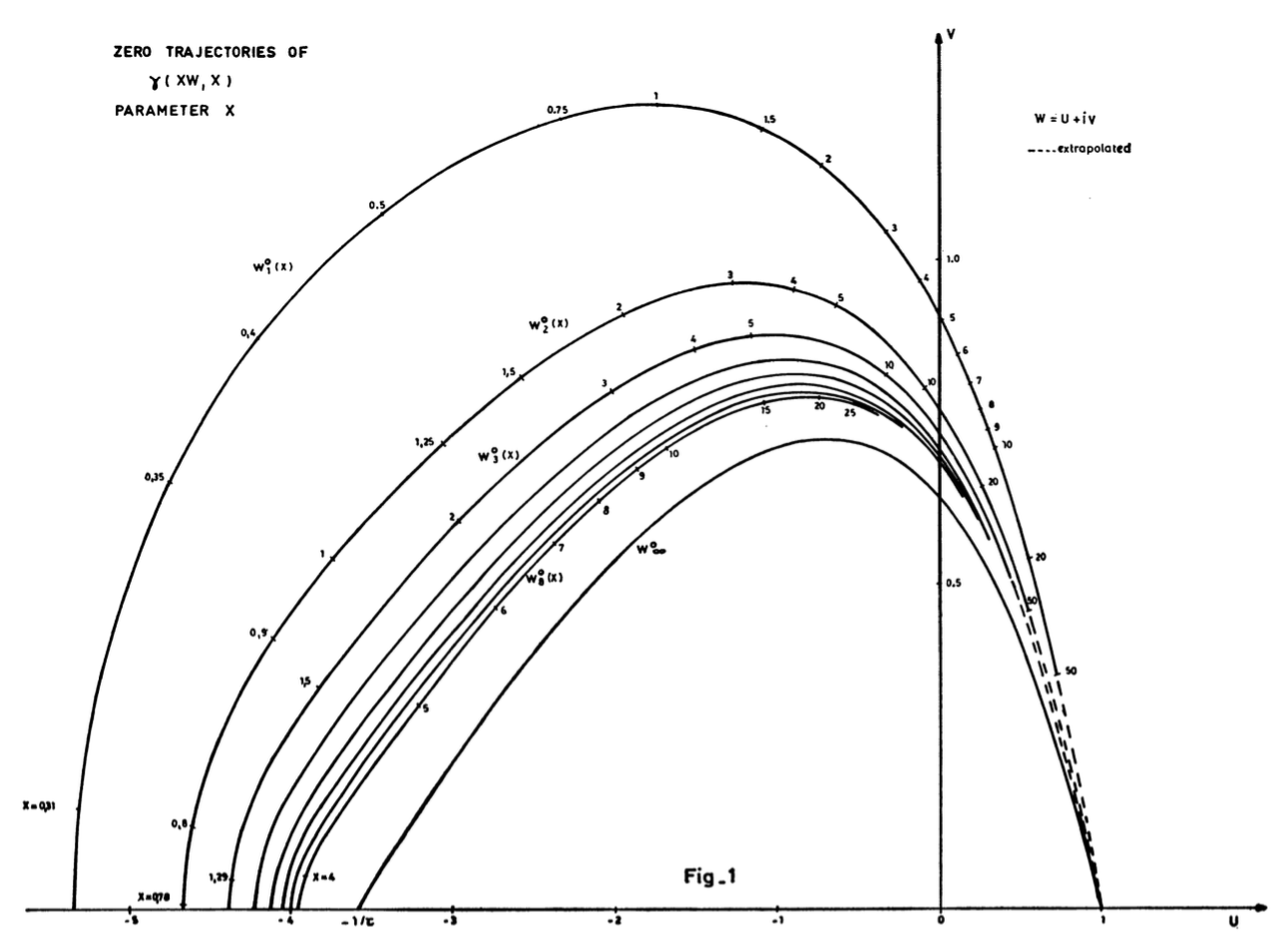# Zeros of the lower incomplete gamma function

I'm interested in the zeros of the lower incomplete gamma function $$\gamma(s,x) = \int_0^x t^{s-1}e^{-t}\,dt \,.$$ In http://www.jstor.org/stable/2007135, Franklin (1919) derives some numerical approximations for the zeros of $\gamma(s,1)$ in terms of $s$, however I've been unable to find any kind of closed form solution or more modern description of these zeros. Does anyone know of any references that discuss the zeros of the lower incomplete gamma function, either in general or at $x=1$?

K.S. Kölbig, On the zeros of the incomplete gamma functions. This paper is from 1972, it has a whole list of references to older literature.

It is the aim of this note to recall some of the earlier results (occasionally correcting them), and to present a plot containing a few of the zero trajectories of the function $$\gamma(xw, x)$$ in the complex $$w$$-plane ($$w = u + iv$$), as functions of the real parameter $$x$$ > 0. It will be seen that these trajectories all lie in a finite region of the $$w$$-plane, and that they cluster towards a limiting curve as shown by Mahler (1930).

This limiting curve is interesting, it is given by $${\rm Re}\,(w\log w-w+1)=0.$$ In the plot below (from Kölbig's paper), you can see how the zeroes approach the limiting curve.• Thank you for the swift and detailed response. I'd looked at this resource a bit, it's how I found Franklin, and it has a wonderful description of the dynamics of the zeros. I was wondering though if any exact results had been derived for the structure of these trajectories, especially in the case $x=1$? Jun 29, 2016 at 21:33
• the most recent paper I know of is from 2012 --- only asymptotics Jun 29, 2016 at 21:45
• @CarloBeenakker, could you please give the title and author of your 2012 ref? I can't access the link. (Many links to papers become broken soon, so I'd suggest always providing the title and the author.) May 1, 2017 at 20:30
• A note on the real zeros of the incomplete gamma function, Ian Thompson May 1, 2017 at 21:45
• Did you see anything on the zeros of the entire function $f(x)=\int_0^1 e^{-xt}t^{s-1}dt=x^{-s}\gamma(s,x)$, its Mellin transform being $\frac{\Gamma(z)}{z-s}$ this function has known-asymptotic on every ray from the origin, on $\Re(x) > 0$ it looks like $x^{-s}$ on $\Re(x)<0$ it looks like $e^{-x}$, thus the zeros get close to the imaginary axis where it is the Fourier transform of $t^{s-1}1_{t\in [0,1]}$ Dec 3, 2019 at 12:49